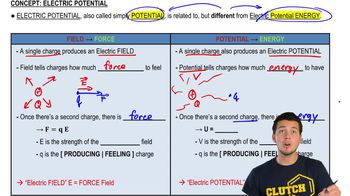Start typing, then use the up and down arrows to select an option from the list.
6:28 minutes
Problem 23e
Textbook Question

# Point charges q_1 = +2.00 μC and q_2 = -2.00 μC are placed at adjacent corners of a square for which the length of each side is 3.00 cm. Point a is at the center of the square, and point bis at the empty corner closest to q_2. Take the electric potential to be zero at a distance far from both charges. (a) What is the electric potential at point a due to q_1 and q_2?Verified Solution
This video solution was recommended by our tutors as helpful for the problem above.
40views